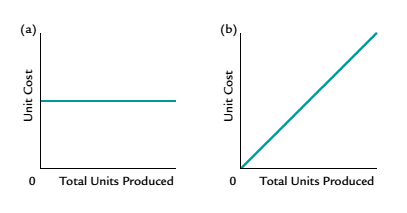Chapter 11, Problem 7CDQ

Chapter
Section
Textbook Problem

Which of the following graphs illustrates how unit variable costs behave with changes in total units produced?To determine

Concept Introduction:

Variable, Fixed and Mixed Cost:

There are three types of costs according to the unit of production; Variable, Fixed and Mixed. Variable costs change proportionally with the number of units produced and variable cost per unit remains constant. Fixed Cost remains same in totality irrespective of the number of units produced. The mixed cost is the mix of variable and fixed cost, some of its part is fixed and some variable.

To Indicate:

The behavior of unit variable costs with change in total units produced

Explanation

There are three types of costs according to the unit of production; Variable, Fixed and Mixed. Variable costs change proportionally with the number of units produced and variable cost per unit remains constant. Fixed Cost remains same in totality irrespective of the number of units produced...

Still sussing out bartleby?

Check out a sample textbook solution.

See a sample solution

The Solution to Your Study Problems

Bartleby provides explanations to thousands of textbook problems written by our experts, many with advanced degrees!

Get Started

Give an example of a future cost that is not relevant.

Managerial Accounting: The Cornerstone of Business Decision-Making

Trace the flow of accounting information through the accounting system.

College Accounting, Chapters 1-27 (New in Accounting from Heintz and Parry)

Explain how payroll fraud can be prevented.

College Accounting (Book Only): A Career Approach

INFLATION Due to a recession, expected inflation this year is only 3%. However, the inflation rate in Year 2 an...

Fundamentals of Financial Management, Concise Edition (with Thomson ONE - Business School Edition, 1 term (6 months) Printed Access Card) (MindTap Course List)

What is a cost object? Give some examples.

Cornerstones of Cost Management (Cornerstones Series)